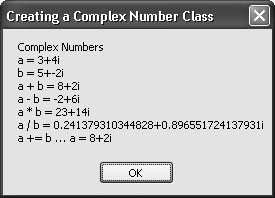# Recipe 6.16. Working with Complex Numbers

#### Problem

You want an easy way to calculate with complex numbers.

#### Solution

Sample code folder: Chapter 06\ComplexNumbers

Create a ComplexNumber structure. Overload the standard mathematical operators so that using complex number variables is easy and natural.

#### Discussion

This recipe provides a great way to see how overloading standard operators can enhance the usability of your classes and structures. In this case, we've created a ComplexNumber structure. Structures are similar to classes, except that they exist as value types rather than reference types. This allows complex number instances to act the same as other simple variables, such as standard numerical variables.

The following code defines the ComplexNumber number structure. Place this code in its own file named ComplexNumber.vb for easy inclusion in any application that requires complex numbers:

` Structure ComplexNumber    Public Real As Double    Public Imaginary As Double        Public Sub New(ByVal realPart As Double, _          ByVal imaginaryPart As Double)       Me.Real = realPart       Me.Imaginary = imaginaryPart    End Sub    Public Sub New(ByVal sourceNumber As ComplexNumber)       Me.Real = sourceNumber.Real       Me.Imaginary = sourceNumber.Imaginary    End Sub    Public Overrides Function ToString() As String       Return Real & "+" & Imaginary & "i"    End Function    Public Shared Operator +(ByVal a As ComplexNumber, _          ByVal b As ComplexNumber) As ComplexNumber       ' ----- Add two   complex numbers together.       Return New ComplexNumber(a.Real + b.Real, _          a.Imaginary + b.Imaginary)    End Operator    Public Shared Operator -(ByVal a As ComplexNumber, _          ByVal b As ComplexNumber) As ComplexNumber       ' ----- Subtract one complex number from another.       Return New ComplexNumber(a.Real - b.Real, _          a.Imaginary - b.Imaginary)    End Operator    Public Shared Operator *(ByVal a As ComplexNumber, _            ByVal b As ComplexNumber) As ComplexNumber       ' ----- Multiply two complex numbers together.       Return New ComplexNumber(a.Real * b.Real - _          a.Imaginary * b.Imaginary, _          a.Real * b.Imaginary + a.Imaginary * b.Real)    End Operator       Public Shared Operator /(ByVal a As ComplexNumber, _          ByVal b As ComplexNumber) As ComplexNumber       ' ----- Divide one complex number by another.       Return a * Reciprocal(b)    End Operator    Public Shared Function Reciprocal( _          ByVal a As ComplexNumber) As ComplexNumber       ' ----- Calculate the reciprocal of a complex number;       '       that is, the 1/x calculation.       Dim divisor As Double          ' ----- Check for divide-by-zero possibility.       divisor = a.Real * a.Real + a.Imaginary * a.Imaginary       If (divisor = 0.0#) Then Throw New DivideByZeroException          ' ----- Perform the operation.       Return New ComplexNumber(a.Real / divisor, _          -a.Imaginary / divisor)    End Function End Structure `

The overloaded New() function lets you instantiate a ComplexNumber number using either a pair of numbers (the real and imaginary parts) or another ComplexNumber number.

The following code demonstrates how complex numbers are created and how standard operators allow mathematical operations such as addition and subtraction in a natural way. The overloaded + operator also impacts the += assignment operator. The last example in the code demonstrates this by adding complex number b to complex number a using the new assignment-operator syntax:

` Dim result As New System.Text.StringBuilder Dim a As ComplexNumber Dim b As ComplexNumber Dim c As ComplexNumber a = New ComplexNumber(3, 4) b = New ComplexNumber(5, -2) c = a + b result.AppendLine("  Complex Numbers") result.AppendLine("a = " & a.ToString()) result.AppendLine("b = " & b.ToString()) ' ----- Addition. c = a + b result.AppendLine("a + b = " & c.ToString()) ' ----- Subtraction. c = a - b result.AppendLine("a - b = " & c.ToString()) ' ----- Multiplication. c = a * b result.AppendLine("a * b = " & c.ToString()) ' ----- Division. c = a / b result.AppendLine("a / b = " & c.ToString()) ' ----- Addition as assignment. a += b result.AppendLine("a += b … a = " & a.ToString()) MsgBox(result.ToString()) `

The ToString() function is overridden in the ComplexNumber structure to format the real and imaginary parts. Figure 6-16 shows the output from the sample code.

##### Figure 6-16. Working with complex numbers in VB 2005Search for " complex numbers" on the Web for more information on this subject.Visual Basic 2005 Cookbook: Solutions for VB 2005 Programmers (Cookbooks (OReilly))
ISBN: 0596101775
EAN: 2147483647
Year: 2006
Pages: 400

Similar book on Amazon